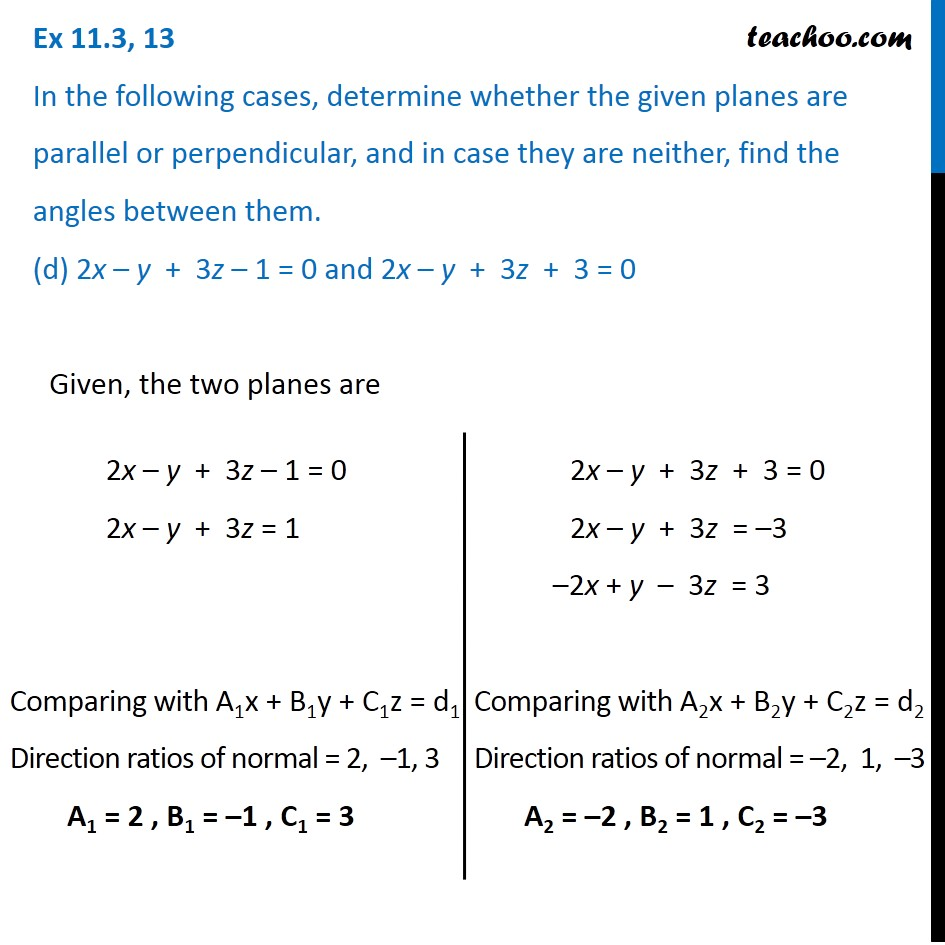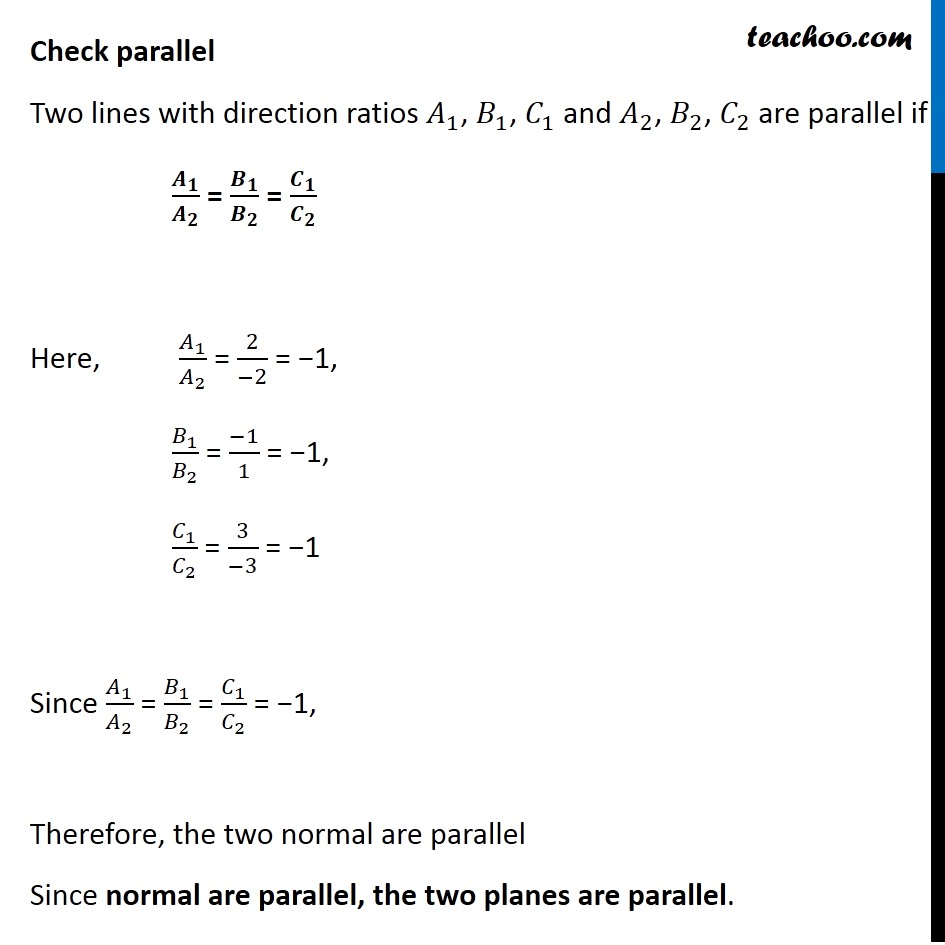Plane

Chapter 11 Class 12 Three Dimensional Geometry
Serial order wiseLearn in your speed, with individual attention - Teachoo Maths 1-on-1 Class

### Transcript

Question 13 In the following cases, determine whether the given planes are parallel or perpendicular, and in case they are neither, find the angles between them. (d) 2x – y + 3z – 1 = 0 and 2x – y + 3z + 3 = 0Given, the two planes are 2x – y + 3z – 1 = 0 2x – y + 3z = 1 Comparing with A1x + B1y + C1z = d1 Direction ratios of normal = 2, –1, 3 A1 = 2 , B1 = –1 , C1 = 3 2x – y + 3z + 3 = 0 2x – y + 3z = –3 –2x + y – 3z = 3 Comparing with A2x + B2y + C2z = d2 Direction ratios of normal = –2, 1, –3 A2 = –2 , B2 = 1 , C2 = –3 Check parallel Two lines with direction ratios 𝐴_1, 𝐵_1, 𝐶_1 and 𝐴_2, 𝐵_2, 𝐶_2 are parallel if 𝑨_𝟏/𝑨_𝟐 = 𝑩_𝟏/𝑩_𝟐 = 𝑪_𝟏/𝑪_𝟐 Here, 𝐴_1/𝐴_2 = 2/(−2) = −1, 𝐵_1/𝐵_2 = (−1)/1 = −1, 𝐶_1/𝐶_2 = 3/(−3) = −1 Since 𝐴_1/𝐴_2 = 𝐵_1/𝐵_2 = 𝐶_1/𝐶_2 = −1, Therefore, the two normal are parallel Since normal are parallel, the two planes are parallel.